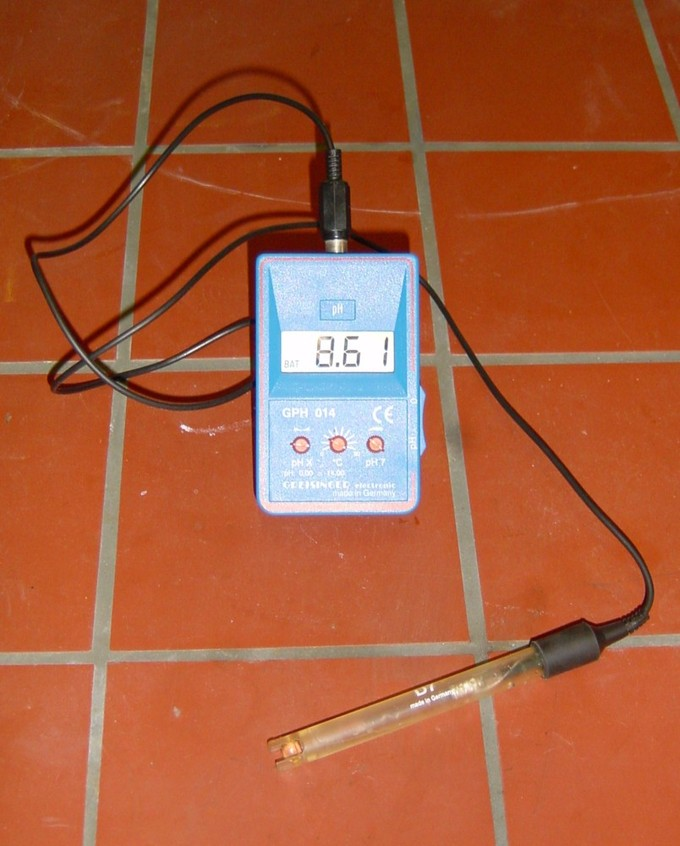## Preparing a Buffer Solution with a Specific pH

#### Learning Objective

• Describe the properties of a buffer solution.

#### Key Points

• Buffer solutions are resistant to pH change because of the presence of an equilibrium between the acid (HA) and its conjugate base (A-).
• When some strong acid is added to a buffer, the equilibrium is shifted to the left, and the hydrogen ion concentration increases by less than expected for the amount of strong acid added.
• Buffer solutions are necessary in biology for keeping the correct pH for proteins to work.
• Buffers can be prepared in multiple ways by creating a solution of an acid and its conjugate base.

#### Terms

• pKaA quantitative measure of the strength of an acid in solution; a weak acid has a pKa value in the approximate range −2 to 12 in water and a strong acid has a pKa value of less than about −2.
• aqueousConsisting mostly of water.
• equilibriumThe state of a reaction in which the rates of the forward (reactant to product) and reverse (product to reactant) reactions are the same.

## Buffers

A buffer is an aqueous solution containing a weak acid and its conjugate base or a weak base and its conjugate acid. A buffer’s pH changes very little when a small amount of strong acid or base is added to it. It is used to prevent any change in the pH of a solution, regardless of solute. Buffer solutions are used as a means of keeping pH at a nearly constant value in a wide variety of chemical applications. For example, blood in the human body is a buffer solution.

Buffer solutions are resistant to pH change because of the presence of an equilibrium between the acid (HA) and its conjugate base (A). The balanced equation for this reaction is:

${ HA\rightleftharpoons H }^{ + }+{ A }^{ - }$

When some strong acid (more H+) is added to an equilibrium mixture of the weak acid and its conjugate base, the equilibrium is shifted to the left, in accordance with Le Chatelier’s principle. This causes the hydrogen ion (H+) concentration to increase by less than the amount expected for the quantity of strong acid added. Similarly, if a strong base is added to the mixture, the hydrogen ion concentration decreases by less than the amount expected for the quantity of base added. This is because the reaction shifts to the right to accommodate for the loss of H+ in the reaction with the base.

Buffer solutions are necessary in a wide range of applications. In biology, they are necessary for keeping the correct pH for proteins to work; if the pH moves outside of a narrow range, the proteins stop working and can fall apart. A buffer of carbonic acid (H2CO3) and bicarbonate (HCO3) is needed in blood plasma to maintain a pH between 7.35 and 7.45. Industrially, buffer solutions are used in fermentation processes and in setting the correct conditions for dyes used in coloring fabrics.

## Preparing a Buffer Solution

There are a couple of ways to prepare a buffer solution of a specific pH. In the first method, prepare a solution with an acid and its conjugate base by dissolving the acid form of the buffer in about 60% of the volume of water required to obtain the final solution volume. Then, measure the pH of the solution using a pH probe. The pH can be adjusted up to the desired value using a strong base like NaOH. If the buffer is made with a base and its conjugate acid, the pH can be adjusted using a strong acid like HCl. Once the pH is correct, dilute the solution to the final desired volume.pH probeThe probe can be inserted into a solution to measure the pH (reading 8.61 in this example). Probes need to be regularly calibrated with solutions of known pH to be accurate.

Alternatively, you can prepare solutions of both the acid form and base form of the solution. Both solutions must contain the same buffer concentration as the concentration of the buffer in the final solution. To get the final buffer, add one solution to the other while monitoring the pH.

In a third method, you can determine the exact amount of acid and conjugate base needed to make a buffer of a certain pH, using the Henderson-Hasselbach equation:

$pH=p{ K }_{ a }+log(\frac { { [A }^{ - }] }{ [HA] } )$

where pH is the concentration of [H+], pKa is the acid dissociation constant, and [A-] and [HA] are concentrations of the conjugate base and starting acid.